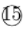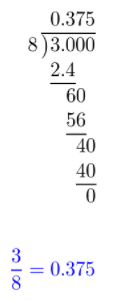# Texas Go Math Grade 7 Module 4 Answer Key Proportionality in Geometry

Refer to our Texas Go Math Grade 7 Answer Key Pdf to score good marks in the exams. Test yourself by practicing the problems from Texas Go Math Grade 7 Module 4 Answer Key Proportionality in Geometry.

## Texas Go Math Grade 7 Module 4 Answer Key Proportionality in Geometry

Write each fraction in simplest form.

Question 1.
$$\frac{25}{30}$$ ___________
Simplify . $$\frac{25}{30}$$
1. List all the factors of the numerator and denominator.
2. Circle the greatest common factor (GCF).
3. Divide the numerator and denominator by the GCF.
25: 1,, 25
30: 1, 2, 3,, 6, 10, 15, 30
$$\frac{25 \div 5}{30 \div 5}$$ = $$\frac{5}{6}$$

Question 2.
$$\frac{27}{36}$$ ___________
Simplify . $$\frac{27}{36}$$
1. List all the factors of the numerator and denominator.
2. Circle the greatest common factor (GCF).
3. Divide the numerator and denominator by the GCF.
27: 1, 3,, 27
36: 1, 2. 3, 4, 6,, 12, 18, 36
$$\frac{27 \div 9}{36 \div 9}$$ = $$\frac{3}{4}$$

Question 3.
$$\frac{14}{19}$$ ___________
Simiplify $$\frac{14}{19}$$
1. List ail the factors of the numerator and denominator.
2. Circle the greatest common factor (GCF).
3. Divide the numerator and denominator by the GCF.
14: 1,, 7, 14
16: 1,, 4, 8, 16
$$\frac{14 \div 2}{16 \div 2}$$ = $$\frac{7}{8}$$

Question 4.
$$\frac{15}{45}$$ ___________
Simplify $$\frac{15}{45}$$
1. List ail the factors of the nuiiiera.tor and denominator.
2. Circle the greatest common factor (GCF).
3. Divide the numerator anti denominator by the GCF.
15: 1. 3, 5,30: 1. 3, 5. 9,, 45
$$\frac{15 \div 15}{45 \div 15}$$ = $$\frac{1}{3}$$

Write each fraction as a decimal.

Question 5.
$$\frac{4}{5}$$ ________
Use long division to write the fraction as a decimal:Question 6.
$$\frac{3}{8}$$ __________
Use long division to write the fraction as a decimal:Question 7.
$$\frac{15}{16}$$ ____________
Use long division to write the fraction as a decimal:Question 8.
$$\frac{13}{20}$$ ____________
Use long division to write the fraction as a decimal:Find the area of each rectangle.

Question 9.
l = 10 cm, w = 4cm __________
A = l × w
A = 10 × 4
A = 40 cm2

Question 10.
l = 14 in., w = 9.5 in. ________
A = l × w
A = 14 × 9.5
A = 133 in.2

Question 11.
l = 0.7cm, w = 0.35cm _______
A = l × w
A = 0.7 × 0.35
A = 0.245 cm2

Question 12.
l = $$\frac{2}{3}$$ yd, w = $$\frac{1}{2}$$ yd ___________
A = l × w
A = $$\frac{2}{3}$$ × $$\frac{1}{2}$$
A = $$\frac{1}{3}$$ yd2

Visualize Vocabulary

Use the ✓ words to complete the graphic. You may put more than one word on each line.Understand Vocabulary

Complete the sentences using the preview words.

Question 1.
What is a proportional two-dimensional drawing of an object? ______________________
A proportional two-dimensional drawing of an object is scale drawing.

Question 2.
What is the distance around a circle? ______________________